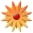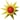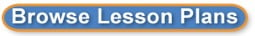# Solving Systems of Linear Equations Using Substitution

Page Views: 6478

Email This Lesson Plan to Me
Overall Rating:(5.0 stars, 1 ratings)

 Subject(s): Grades 7 through 12 NETS-S Standard: Creativity and InnovationCommunication and CollaborationCritical Thinking, Problem Solving, and Decision MakingDigital CitizenshipTechnology Operations and ConceptsView Full Text of Standards School: Galax High School, Galax, VA Planned By: Eric Tatum Original Author: Eric Tatum, Galax
LESSON PREPARATION (BEFORE):

First you will need to create a notebook file using the SMART Notebook software with which SMART Boards work. The notebook file will contain example problems that you will use to introduce your students to the process of solving a system of equations with substitution. A basic system of equations that you could use as your first example problem would be x = 2y - 2 and x + 2y = 2.
I recommend making each successive problem a little more difficult than the last, ending with a problem as difficult as (1/2)x - 2y = -4 and 3x - (1/3)y = 1.
Once you have a notebook file with slides of example problems to be worked using substitution, you are ready to go!

THE LESSON (DURING):

Normally, when students begin solving systems of equations, they do it first by graphing them and finding the intersection of the two lines, which is the solution. As an introduction to solving using substitution, review solving by graphing. I suggest having students solve the first problem from your notebook file (in my case x = 2y -2 and x + 2y = 2) by graphing it to find the intersection. This can be done using pencil and paper, or on a graphing calculator. Encourage your students to collaborate while solving the problem. Facilitate by walking around the room, offering suggestions and help where needed. Students should find that the solution to the system is x = 0 and y = 1, or the ordered pair (0,1). Emphasize to students that the solution to a system is always an x-value AND a y-value. Both values are needed for a complete solution. This will be useful later when solving systems using substitution. Now pique your students' interests by posing the following question. "What if there was a way to find the solution without graphing?" Let any discussion occur, and then tell the students that the goal of today's lesson is to do just that--solve a system of equations without graphing to find the intersection of two lines.

Explain that you are going to work the same problem with the students again, except in a different way this time. Pose the following question. "What do you think it means in the first equation when it reads that x equals 2y - 1?" The answer that you are looking for is that x is equivalent or x is the same thing as 2y - 1. The two expressions just look different. Explain to the students that since the two expressions are the same, replacing (or substituting) one for the other won't change anything. This means that the x in the second equation is really the same thing as 2y - 1, so we can replace (or substitute) the x with 2y - 1.

This is usually the point where many students cannot connect the previous verbal statement with what they are actually supposed to do. However, using the SMART Board, you can show them exactly what happens. You can use the SMART Board to select the 2y - 1 and physically drag it over top of the x in the second equation, thus replacing (or substituting) x with 2y - 1. The physical movement of the 2y - 1 over the x is much more meaningful to students who are concrete thinkers, and it reinforces the idea for students who can think more abstractly.

Once you have performed the substitution, explain to the students that since your new equation only has one variable (in this case, y), you can solve the equation for it. Solve the equation with your students to get y = 1. Remind the students again how a complete solution has two values, and now we must find the x-value.
Now we are going to substitute again. Since y = 1, we can replace y with 1 in either of the originial equations. Using the SMART Board, drag the 1 up to either of the originial equations and put it on top of y, reminding the students that the 2 in front of y means multiplication. The students can now solve this equation for x, getting the solution x = 0. Remind the students that the x-value and y-value can be written as the ordered pair (0,1), just like when they solved the system by graphing.

Address any questions that the students have, and proceed to working on the rest of the example problems, using the SMART Board for substitution in each problem. I usually like to have a student "play teacher" and work through the next problem on the board with the students like I just did.

LESSON REFLECTION (AFTER):

Reflect on what you will do differently the next time you present this lesson. Think about what examples work better than others for showing students the process of substitution. Evaluate the key points of the lesson that you need to emphasize more for next time. Focus on posing questions to students that promote higher-level thinking. I always encourage my students to give me feedback after a lesson by asking the questions, "How would you have taught this lesson to your friends? Should I have add more to the lesson, or should I have left some of it out?"

 Follow-Up This lesson serves as a good introduction to solving systems of equations by elimination, and the SMART Board can also be used to provide a physical sense of "eliminating" a variable from a system of equations. Materials: Graphing, Calculators, Projectors, Whiteboards Other Items: 1 PC or Apple Computer